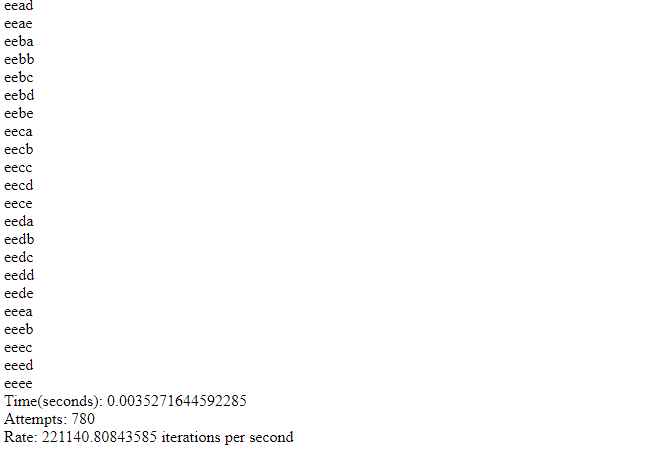# Sample Code for Algorithm to Create Combinations of Characters

In my post here, I described an algorithm to create all possible combinations from a set of characters. However, it allows repetitions of characters in the generated combinations. e.g aas, aaa and so on.
In this post, I will demonstrate an implementation of this algorithm in PHP, without any parallelization of any kind. I don’t know if it’s even possible to parallelize code in PHP.

You can run this code on LAMPP, WAMP, MAMP or XAMPP if you like. Successive trials of code execution may be faster than previous ones. Maybe, you all won’t like this code since it uses the “goto” keyword which is believed to lead to the creation of spaghetti code.  However, this was the easiest way to implement the algo for me.

```<?php

\$dictionary = array('a', 'b', 'c', 'd', 'e');

\$startLen = 1;
\$stopLen = 4;

\$currLen = 1;

//function to create string combination from array of indices
function getStringFromChars(\$indices)
{
global \$dictionary;
\$str='';

for(\$j = 0; \$j < count(\$indices); \$j++)
{
\$str.=\$dictionary[\$indices[\$j]];
}

return \$str;
}

//check condition to stop generating combos
function timeToStop(\$indices)
{

global \$dictionary;

\$stop = false;

for(\$j = 0; \$j < count(\$indices); \$j++)
{
if(\$indices[\$j] < count(\$dictionary) - 1)
{
return \$stop;
}
}

\$stop = true;

return \$stop;
}

//main function
function generate(\$startLen, \$stopLen)
{
global \$dictionary;
\$attempts = 0;

for(\$currLen = \$startLen; \$currLen <= \$stopLen; \$currLen++)
{
step3:
\$chars = array();

\$indices = array();

//init the arrays
for(\$i = 0; \$i < \$currLen; \$i++ )
{
\$indices[\$i] = 0;

\$chars[\$i] = \$dictionary[\$indices[\$i]];
}

step4:
\$working_index = \$currLen - 1;

step5:
\$str = getStringFromChars(\$indices);

step6:
echo \$str;
echo "<br />";
\$attempts++;

if(timeToStop(\$indices))
{
if(\$currLen < \$stopLen)
{

continue;
}
else
{
break;
}
}

if(\$indices[\$working_index] < count(\$dictionary) - 1)
{
\$indices[\$working_index]++;
goto step5;
}
else
{
if(\$currLen > 1)
{

while(\$indices[\$working_index] == count(\$dictionary) - 1)
\$working_index--;

if(\$working_index == -1)
{
if(\$currLen < \$stopLen)
{

continue;
}
else
break;
}
else
{
\$indices[\$working_index]++;
//set all indices to the right of working_index to 0

for(\$k = \$working_index + 1; \$k < count(\$indices); \$k++)
{
\$indices[\$k] = 0;
}

goto step4;
}
}
else
{

if(\$currLen < \$stopLen)
{

continue;
}
else
break;
}
}

}

return \$attempts;
}

\$start = microtime(true);

\$atts = generate(\$startLen, \$stopLen);

\$end = microtime(true);

\$time_taken = \$end - \$start;

\$rate = \$atts / \$time_taken;

echo "Time(seconds): ".\$time_taken;
echo "<br />";

echo "Attempts: ".\$atts;
echo "<br />";

echo "Rate: ".\$rate." iterations per second";
```